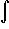#Interactive Real Analysis

Next | Previous | Glossary | Map

## 7.4. Lebesgue Integral

### Definition 7.4.19: The General Lebesgue Integral

Let f be a measurable function and define the positive and negative parts of f, respectively, as:
f +(x) = max(f(x), 0)
f -(x) = max(-f(x), 0)
so that f = f + - f -. Then f is Lebesgue integral if f + and f - are Lebesgue integrable andE f(x) dx =E f +(x) dx -E f -(x) dx =
Next | Previous | Glossary | Map